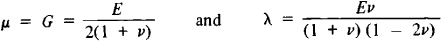# Lamé Constants

(redirected from Lame constants)

## Lamé constants

[lä′mā ‚kän·stəns]
(mechanics)
Two constants which relate stress to strain in an isotropic, elastic material.

## Lamé Constants

values that relate the components of elastic stress at some point of a solid isotropic deformable body to the components of strain at this point:

σx = 2μεxx + λ(εxx + εyy + εzz)

τ = μεxy

where σ and τ are the normal and tangential components of stress, ε are the components of strain, and the coefficients λ and μ are Lamé constants, named after the French mathematician G. Lamé. The Lamé constants depend on the material and its temperature. They are related to the modulus of elasticity (Young’s modulus) and Poisson’s ratio v bywhere E is the modulus of elasticity and G is the shear modulus.

References in periodicals archive ?
and the spacetime continuum Lame constants are as per (28) and (29):
The values for the Lame constants and the third-order elastic constants are approximated on the basis of the experimental data ; as a result the linear elastic constant is [[alpha].
1/2] is the effective stress, I is the identity tensor, [lambda] and [mu] are the Lame constants related to the Young's modulus E and the Poisson's ratio v, and H' is strain hardening parameter.
Generally, the memory function has the same form as Eq 30, with the Lame constants or the Young's modulus sometimes used in place of the shear modulus.
2] are the memory functions associated with the Lame constants, represented as
p] are the Lame constants of the pth Maxwell element and [[xi].
We consider time-harmonic elastic waves with circular frequency [omega] and it will be assumed that all Lame constants and the frequency are positive.
where [lambda] and [mu] are the Lame constants, H' strain hardening parameter, [sigma]' is the deviatoric Cauchy stress tensor, [[sigma].
Site: Follow: Share:
Open / Close